# Hamilton operator

(diff) ← Older revision | Latest revision (diff) | Newer revision → (diff)

nabla operator,-operator, Hamiltonian

A symbolic first-order differential operator, used for the notation of one of the principal differential operations of vector analysis. In a rectangular Cartesian coordinate system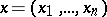with unit vectors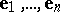, the Hamilton operator has the formThe application of the Hamilton operator to a scalar function, which is understood as multiplication of the "vector"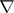by the scalar, yields the gradient of: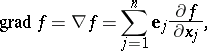i.e. the vector with components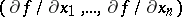.

The scalar product ofwith a field vector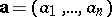yields the divergence of: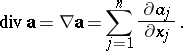The vector product ofwith the vectors,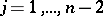, yields the curl (rotation, abbreviated by rot) of the fields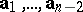, i.e. the vector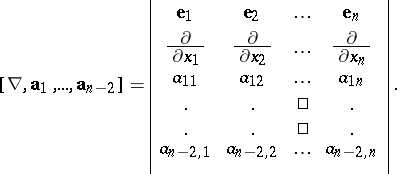If,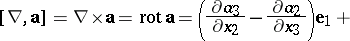The scalar square of the Hamilton operator yields the Laplace operator: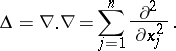The following relations are valid: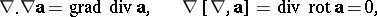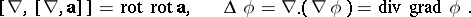The Hamilton operator was introduced by W. Hamilton .

How to Cite This Entry:
Hamilton operator. Encyclopedia of Mathematics. URL: http://encyclopediaofmath.org/index.php?title=Hamilton_operator&oldid=11494
This article was adapted from an original article by L.P. Kuptsov (originator), which appeared in Encyclopedia of Mathematics - ISBN 1402006098. See original article